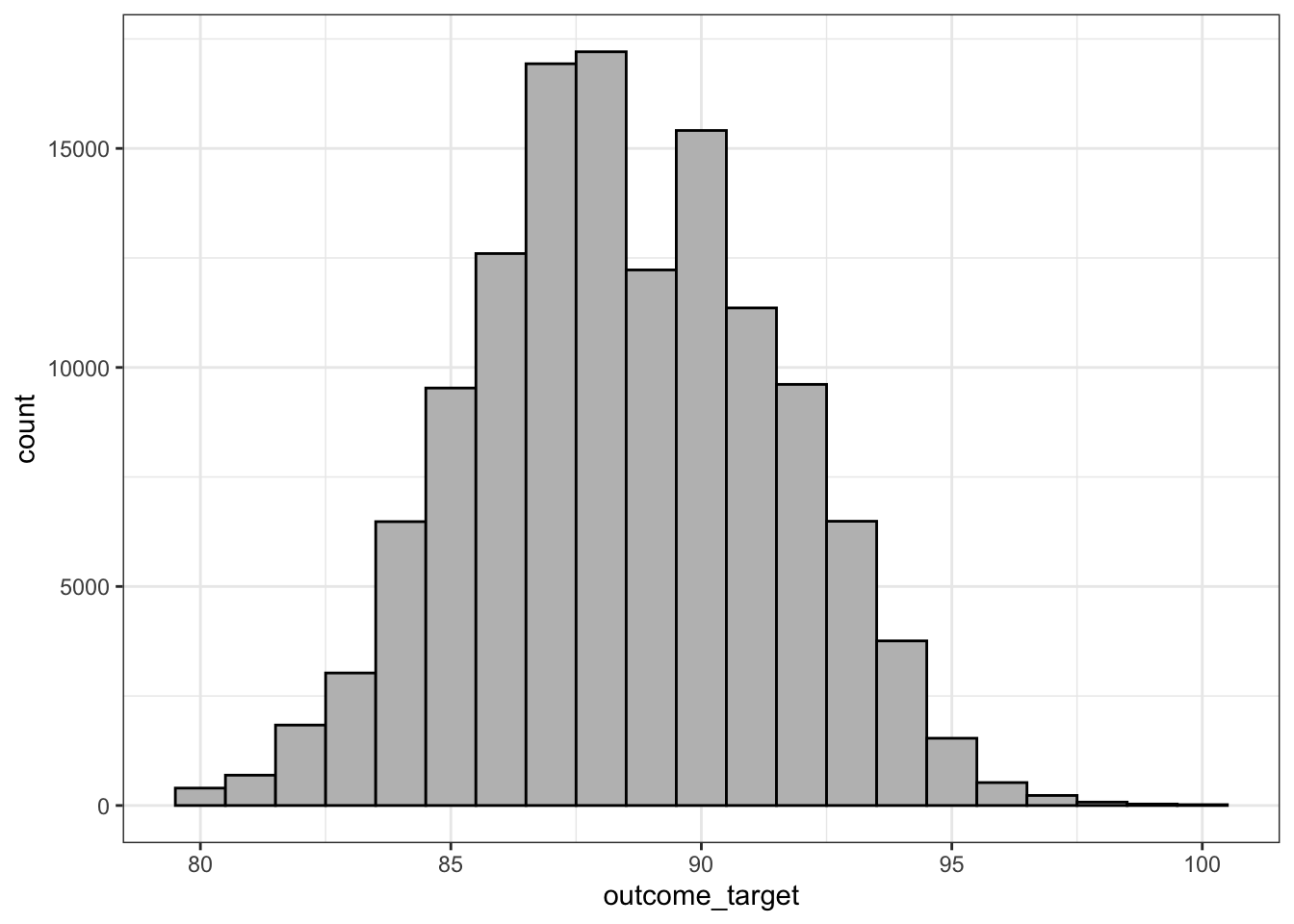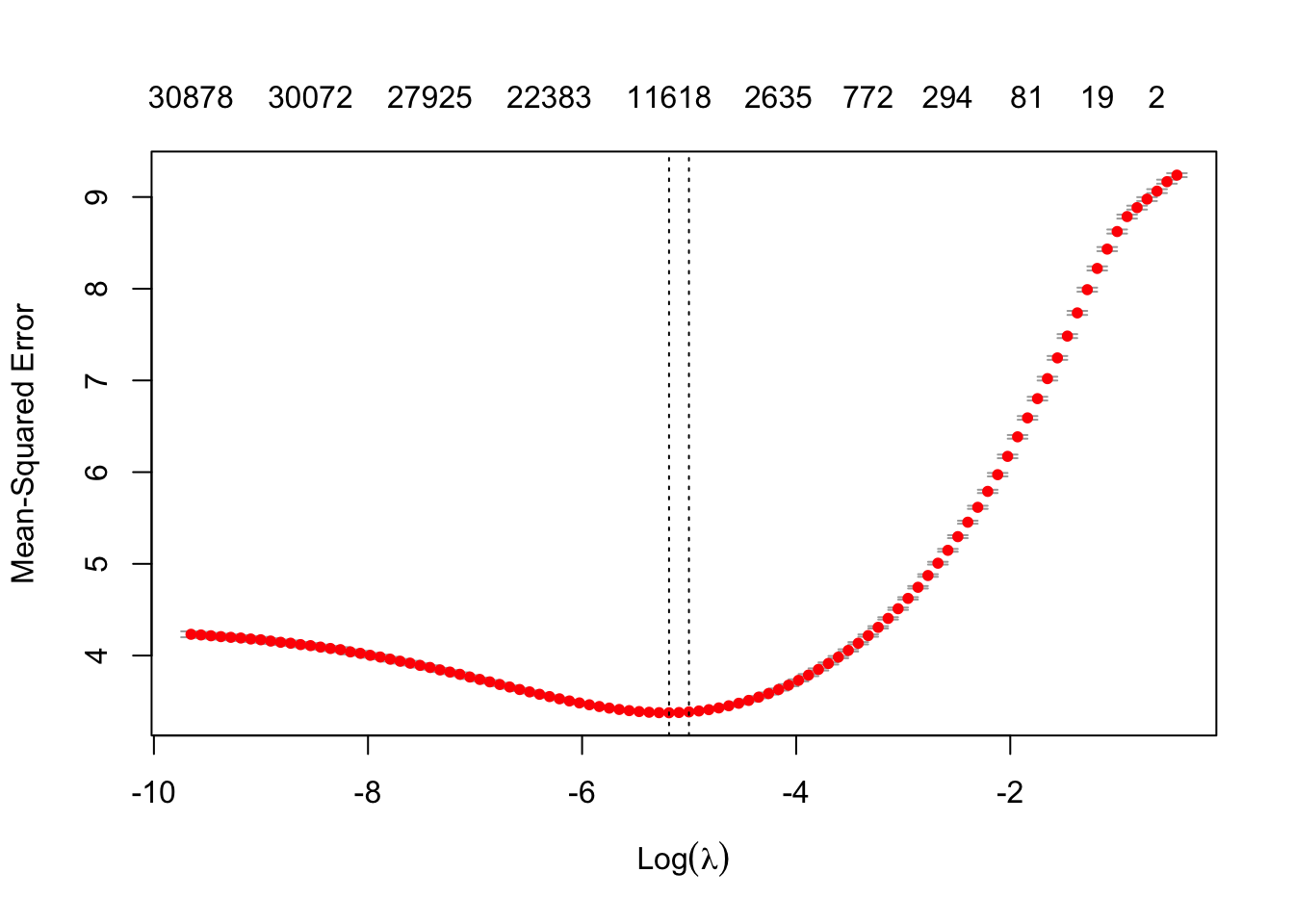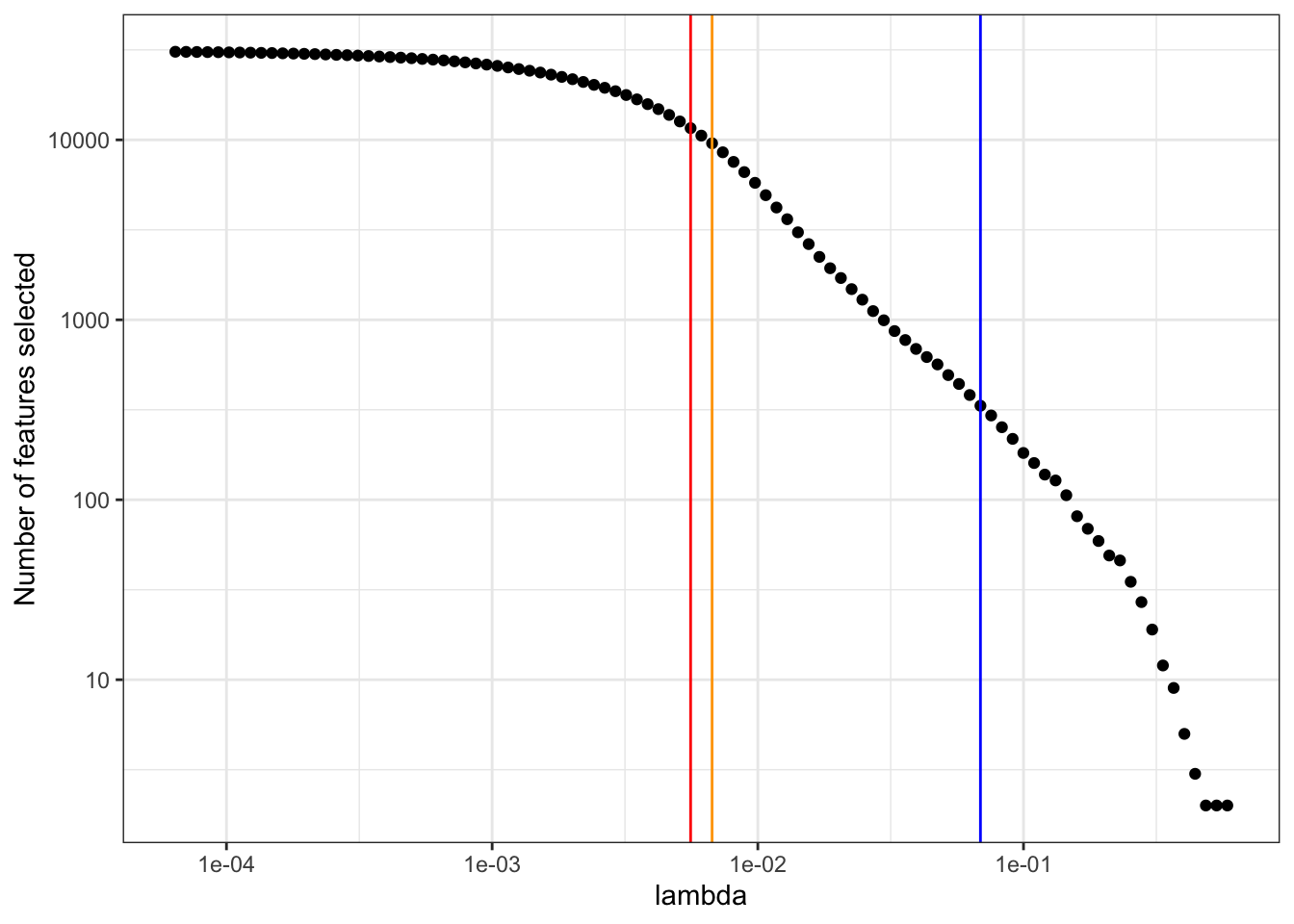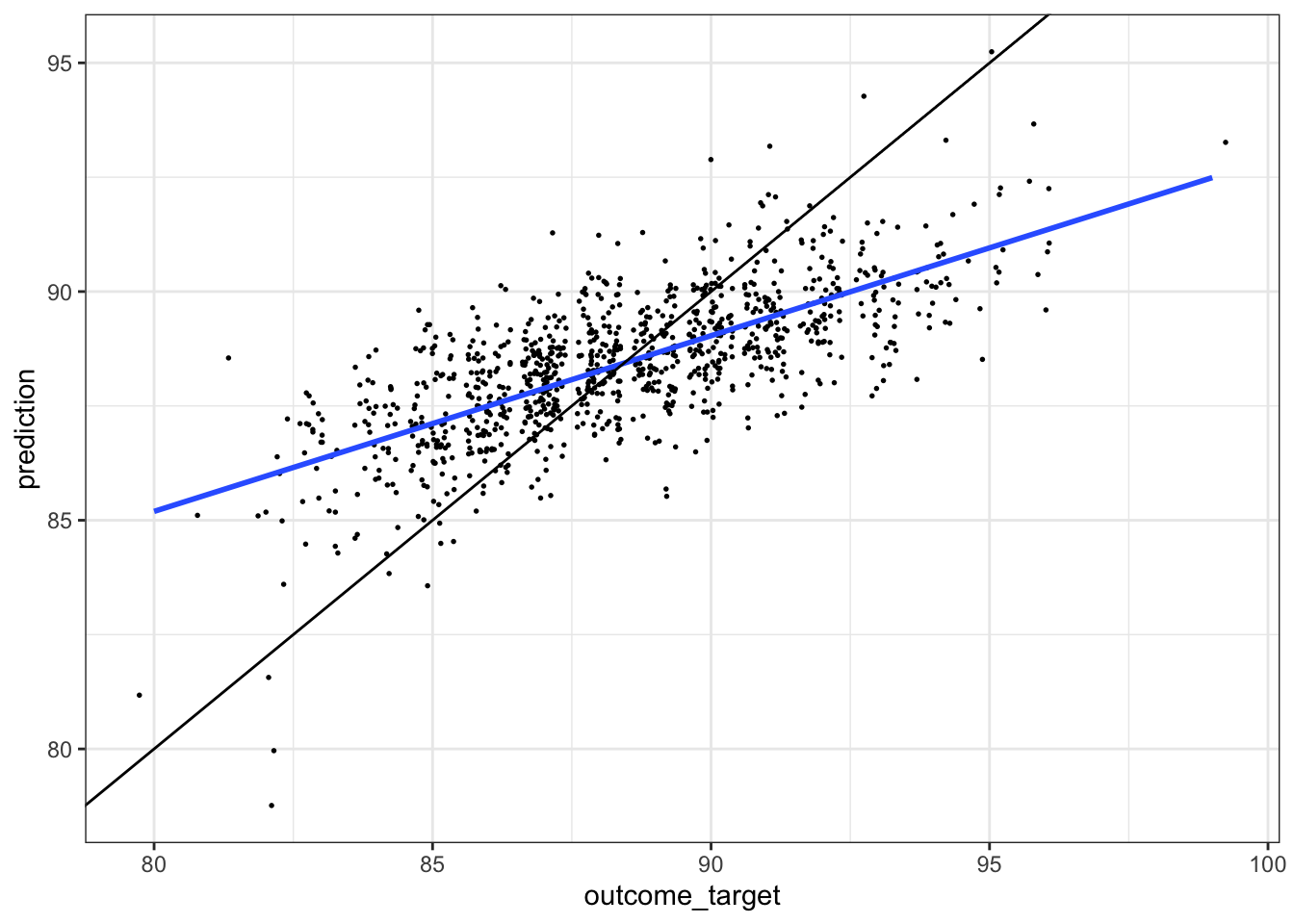# Tidy Tuesday – NLP on Wine Ratings Dataset

Natural language processing of wine descriptions to predict wine review scores.
R
Machine learning
Published

May 28, 2019

I attended a Greater Boston useR Group (R Programming Language) Meetup yesterday, where the topic was to work on a Tidy Tuesday dataset. This week was a wine-enthusiast ratings dataset from Kaggle.

I adapted some code I had previously written for a clinical dataset to analyze the text of the wine descriptions to predict wine scores. There was some interest in seeing the code I used, so I’m posting it here. I tried to include some explanatory comments in the code.

Would you rather have wine described with words like: “stunning, gorgeous, long, complex, beautiful, beautifully, superb, impressive, vineyard, years, beauty, decade, delicious, velvety, powerful”?

Or instead, with: “aromas, easy, tastes, sour, strange, lean, rustic, short, flat, bitter, thin, lacks, soft, simple, flavors”?

These were some of the positive and negative features identified.

## Overall approach:

• use `tidytext` to tokenize all the words and generate term frequency-inverse document frequency (TF-IDF) scores for each word in each wine description
• the predictor matrix ends up pretty large, with 129,971 rows (wines) and ~31,000 prediction features (unique words)
• use `glmnet` to generate a linear regression model to predict wine scores, using LASSO (L1) regularization to perform feature selection on the word features, and cross-validation to identify an appropriate regularization strength to result in a reasonable number of word features

This would also be an easy way to identify useful word features for sentiment analysis of wine reviews.

# Predict point score by regularized linear regression using TF-IDF of descriptions

``````library(tidyverse) # https://www.tidyverse.org; https://r4ds.had.co.nz
library(tidytext)  # Julia Silge and David Robinson; https://www.tidytextmining.com
library(glmnet)    # Jerome Friedman et al; https://cran.r-project.org/web/packages/glmnet/glmnet.pdf

seed <- 1           # random seed called before glmnet cross-validation
num_features <- 300 # set approximate number of (word) features to select, via lambda for regularization``````
``````# Tidy Tuesday: https://github.com/rfordatascience/tidytuesday
# - 2019-05-28 dataset: https://github.com/rfordatascience/tidytuesday/tree/master/data/2019/2019-05-28
# - dataset from: https://www.kaggle.com/zynicide/wine-reviews
wine <- read.csv("winemag-data-130k-v2.csv", stringsAsFactors = FALSE)
names(wine) <- 'id' # give the unique identifier column a name``````

## Prep dataset

Simplify dataset to dataframe with only 3 columns:

• `id`: unique id
• `text`: source of text
• `outcome_target`: outcome target to predict
``````df <- wine %>%
transmute(
id = as.character(id),  # will use for table joins
text = description,     # source of text
outcome_target = points # define the outcome target: points + price for continuous, but price is very skewed
) %>%
filter(!is.na(outcome_target)) # glmnet will fail if any missing values

# df <- df %>% sample_n(1500) # if don't want to train on full 129,000+ wine dataset...``````

## Distribution of outcome target

``df %>% ggplot(aes(outcome_target)) + geom_histogram(color = 'black', fill = 'grey', binwidth = 1) + theme_bw()``## Tidy text

• Tokenize the text to lowercase words, remove numbers, remove stopwords
• Output is for each unique id, the count of each word feature
``````tidy <- df %>%
select(id, text) %>%
unnest_tokens(word, text) %>% # tidytext tokenize text to lowercase words
mutate(word = str_extract(word, "[a-z']+")) %>% # greedy matches first string of [a-z] of length at least 1, removing trailing non-letters
filter(!is.na(word)) %>% # all those tokens which had NO [a-z] characters, like numbers
anti_join(get_stopwords(), by = 'word') %>% # remove stopwords
count(id, word, sort = TRUE)``````
``head(tidy) ## Count of each unique word token for each id``
``````      id   word n
1  54963  black 6
2 106687  white 5
3  11616  white 5
4 127916 cherry 5
5  20435   wine 5
6  34198  white 5``````

## What are the most common words in the entire corpus?

``tidy %>% group_by(word) %>% summarise(n = sum(n)) %>% arrange(desc(n)) %>% head()``
``````# A tibble: 6 × 2
word        n
<chr>   <int>
1 wine    78337
2 flavors 62795
3 fruit   49928
4 aromas  39639
5 palate  38437
6 acidity 35003``````

## Calculate tf, idf, and tf-idf values

``````tidy_tf_idf <- tidy %>%
bind_tf_idf(word, id, n) # for each id / word, calculate n, tf, idf, tf_idf

``````      id   word n        tf       idf    tf_idf
1  54963  black 6 0.1538462 1.6700270 0.2569272
2 106687  white 5 0.1282051 2.4124344 0.3092865
3  11616  white 5 0.2173913 2.4124344 0.5244423
4 127916 cherry 5 0.2173913 1.5563670 0.3383406
5  20435   wine 5 0.1388889 0.7209121 0.1001267
6  34198  white 5 0.2173913 2.4124344 0.5244423``````

## Add rows which contain the outcome target

``````# - a little kludgy -- will force the outcomes into the same 3 columns as tidy_tf_idf's, so can bind_rows
# - for each 'id', the 'word' will be 'outcome_target' and the 'tf_idf' will be the prediction target
# - will later pull this out as the vector 'y' prediction target
tidy_tf_idf <- tidy_tf_idf %>%
bind_rows(
df %>% transmute(
id = id,
word = 'outcome_target',
tf_idf = outcome_target
)
)``````

## Convert from tidy long format to sparse matrix and prediction target vector for glmnet

• each row is a unique wine: n = 129,971
• each column is a word feature: n = tens of thousands
• this is a huge matrix – since word features are sparse, will cast to a sparse matrix
``````# Cast to sparse matrix
sp <- cast_sparse(tidy_tf_idf, row = id, column = word, value = tf_idf)
sp <- sp[, c(which(colnames(sp)=="outcome_target"), which(colnames(sp) != "outcome_target"))] # move 'outcome_target' to first column
dimnames(sp)[] %>% head # confirm column names, with 'outcome_target' moved to first column; each column is a unique predictor word token``````
`````` "outcome_target" "black"          "white"          "cherry"
 "wine"           "orange"        ``````
``````x <- sp[, 2:dim(sp)]  # predictor matrix, all but the first column
y <- as.numeric(sp[, 1]) # outcome vector of point scores, from the first column

dim(x) # sparse matrix of dimensions: # of documents x # of unique word tokens``````
`` 129971  31725``

## glmnet elasticnet model building, using cross-validation to choose regularization strength

• primarily LASSO regularization, to get feature selection and not just coefficient shrinkage
``````##### Fit glmnet elasticnet model #####
set.seed(seed)
# degree of L1 versus L2 regularization -- glmnet: alpha = 0.99 'performed much like the lasso, but removes any degeneracies ... caused by extreme correlations'
cvfit = cv.glmnet(x, y, type.measure = "mse", alpha = 0.99, nfolds = 10) # primarily LASSO, to get feature selection
# cvfit = cv.glmnet(x, y, family = "binomial", type.measure = "auc", alpha = 0.99, nfolds = 20) # if wanted binary classification, logistic regression, maximizing AUC``````

## Result of search for optimal lambda for regularization

• on top of plot, show numbers of non-zero coeffecients (i.e., number of word predictors selected)
``````# Not going to use lambdas that minimize the cost function, because with the wine dataset, selects too many features
# cvfit\$lambda.min # lambda resulting minimizing cost function
# cvfit\$lambda.1se # typically use 1 standard error from minimum
plot(cvfit) # cross-validation search for optimal lambda````````````# create a dataframe of tested lambdas, number of predictors, and list of predictors at each lambda
len = length(cvfit\$lambda)
predictors_select <- tibble(lambda = cvfit\$lambda, nzero = cvfit\$nzero, features = vector('character', len))
for (i in 1:len) {
index_list <- which(coef(cvfit, s = predictors_select\$lambda[i]) != 0) # indices of the predictor columns to keep
temp <- tibble( # features by decreasing magnitude; outcome_target is the intercept term
word = colnames(sp)[index_list],
beta = coef(cvfit, s = cvfit\$lambda[i])[index_list]
) %>%
arrange(desc(abs(beta)))
predictors_select\$features[i] <- paste(temp\$word, collapse = '#')
}``````

## Choose a lambda regularization strength that generates a desired approximate number of selected features

``````lambda_num_features <- predictors_select %>% # find the maximum lambda that generates at least num_features features
arrange(desc(lambda)) %>%
filter(nzero >= num_features) %>%
.\$lambda %>%
max()

lambda_num_features``````
`` 0.0688468``

## Plot of number of predictors selected versus increasing regularization lambda

``````predictors_select %>%
filter(nzero != 0) %>%
ggplot(aes(lambda, nzero)) +
geom_point() +
geom_vline(xintercept = cvfit\$lambda.min, color = 'red') +
geom_vline(xintercept = cvfit\$lambda.1se, color = 'orange') +
geom_vline(xintercept = lambda_num_features, color = 'blue') +
scale_x_continuous(trans='log10') +
scale_y_continuous(trans='log10') +
labs(y = 'Number of features selected') +
theme_bw()``````## Generate a dataframe that fully describes the model

• contains the word features selected, the beta coefficients, and the inverse document frequency of that term within the training corpus
• (if wanted to do predictions on new data, would need to normalize the term frequencies to the IDF of the training corpus)
``````optimal_lambda <- lambda_num_features

# create data frame of predictors, coefficients, and idf values, sorted by decreasing coefficient
indices <- which(coef(cvfit, s = optimal_lambda) != 0) # indices of the predictor columns to keep
predictors <- tibble( # display predictors by decreasing magnitude; outcome_target is the intercept term
word = colnames(sp)[indices],
beta = coef(cvfit, s = optimal_lambda)[indices]
) %>%
left_join( # Get the IDF's for the chosen terms, to be able to apply model to future datasets
tidy_tf_idf %>% filter(word %in% colnames(sp)[indices]) %>% select(word, idf) %>% unique,
by = 'word'
) %>%
arrange(desc(beta))``````

## Predictors with the most positive coefficients (associated with a high outcome_target)

``head(predictors %>% filter(word != 'outcome_target'), n = 10)``
``````# A tibble: 10 × 3
word         beta   idf
<chr>       <dbl> <dbl>
1 stunning     9.99  6.13
2 gorgeous     8.84  5.26
3 long         8.35  3.02
4 complex      8.25  3.32
5 beautiful    7.99  4.43
6 beautifully  7.21  4.51
7 superb       7.14  6.18
8 impressive   6.92  4.26
9 vineyard     6.75  3.10
10 years        6.33  2.85``````

## Predictors with the most negative coefficients (associated with a low outcome_target)

``tail(predictors, n = 10)``
``````# A tibble: 10 × 3
word      beta   idf
<chr>    <dbl> <dbl>
1 lean     -4.67 4.02
2 rustic   -4.69 4.57
3 short    -4.76 4.60
4 flat     -5.03 5.08
5 bitter   -5.41 3.69
6 thin     -5.47 4.94
7 lacks    -5.78 5.04
8 soft     -5.91 2.28
9 simple   -7.92 3.53
10 flavors -13.8  0.779``````

## Make predictions

``pred <- predict(cvfit, s = optimal_lambda, newx = x)``

## Root mean square error of predictions

``````df <- df %>%
left_join(
tibble(id = rownames(pred), prediction = pred[,1]), # add column of predictions
by = 'id'
)

sqrt(mean((df\$outcome_target - df\$prediction)^2))``````
`` 2.231615``

## Plot of predictions versus actual target

• the black line would represent perfect prediction
• the lower slope of the model (blue line) suggests an underestimate of prediction at higher target values
• not sure why the slope isn’t one
``````df %>%
sample_n(1000) %>%
ggplot(aes(outcome_target, prediction)) +
geom_jitter(size = 0.3) +
geom_smooth(method = 'lm', se = FALSE) +
geom_abline(slope = 1, intercept = 0) +
theme_bw()``````
```geom_smooth()` using formula 'y ~ x'``## Other thoughts

• do different varieties of wine have different word features? E.g., perhaps ‘sweet’ might be good for a dessert wine, but not so good for a red wine?
• do different reviewers systematically use words differently?
• is there a good way to combine other features with the TF-IDF matrix of description text, to improve model performance?
• (even with this TF-IDF model, the cross validation suggests using ~10,000 word features would improve performance, without overfitting, but that seems unlikely)
• to predict on new data, need to use IDF scores of the training corpus, and need additional code to create a feature matrix that includes all word features of the model, even if not present in the new data